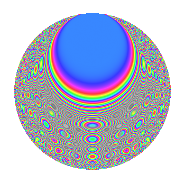# Properties

 Label 504.2.brLevel 504 Weight 2 Character orbit br Rep. character $$\chi_{504}(155,\cdot)$$ Character field $$\Q(\zeta_{6})$$ Dimension 144 Newform subspaces 1 Sturm bound 192 Trace bound 0

# Related objects

## Defining parameters

 Level: $$N$$ = $$504 = 2^{3} \cdot 3^{2} \cdot 7$$ Weight: $$k$$ = $$2$$ Character orbit: $$[\chi]$$ = 504.br (of order $$6$$ and degree $$2$$) Character conductor: $$\operatorname{cond}(\chi)$$ = $$72$$ Character field: $$\Q(\zeta_{6})$$ Newform subspaces: $$1$$ Sturm bound: $$192$$ Trace bound: $$0$$

## Dimensions

The following table gives the dimensions of various subspaces of $$M_{2}(504, [\chi])$$.

Total New Old
Modular forms 200 144 56
Cusp forms 184 144 40
Eisenstein series 16 0 16

## Trace form

 $$144q + 6q^{6} + O(q^{10})$$ $$144q + 6q^{6} - 12q^{12} + 34q^{18} - 42q^{20} - 30q^{24} - 72q^{25} + 24q^{27} - 36q^{30} - 30q^{32} - 16q^{33} + 12q^{34} - 12q^{36} + 12q^{40} + 24q^{41} - 20q^{42} - 24q^{46} - 24q^{48} + 72q^{49} - 78q^{50} + 18q^{52} + 10q^{54} + 8q^{57} - 18q^{58} - 72q^{59} + 88q^{60} - 12q^{64} + 2q^{66} + 78q^{68} + 42q^{72} + 84q^{74} - 112q^{75} + 12q^{76} + 112q^{78} - 8q^{81} - 36q^{82} - 14q^{84} + 30q^{86} + 24q^{88} + 104q^{90} - 114q^{92} - 42q^{94} - 80q^{96} - 64q^{99} + O(q^{100})$$

## Decomposition of $$S_{2}^{\mathrm{new}}(504, [\chi])$$ into newform subspaces

Label Dim. $$A$$ Field CM Traces $q$-expansion
$$a_2$$ $$a_3$$ $$a_5$$ $$a_7$$
504.2.br.a $$144$$ $$4.024$$ None $$0$$ $$0$$ $$0$$ $$0$$

## Decomposition of $$S_{2}^{\mathrm{old}}(504, [\chi])$$ into lower level spaces

$$S_{2}^{\mathrm{old}}(504, [\chi]) \cong$$ $$S_{2}^{\mathrm{new}}(72, [\chi])$$$$^{\oplus 2}$$

## Hecke Characteristic Polynomials

There are no characteristic polynomials of Hecke operators in the database